Get inspired by the success stories of our students in IIT JAM MS, ISI  MStat, CMI MSc DS.  Learn More

# ISI MStat PSB 2013 Problem 7 | Bernoulli interferes NormallyThis is a very simple and beautiful sample problem from ISI MStat PSB 2013 Problem 7. It is mainly based on simple hypothesis testing of normal variables where it is just modified with a bernoulli random variable. Try it!

## Problem- ISI MStat PSB 2013 Problem 7

Suppose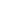and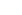are two independent and identically distributed random variables with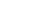. Further consider a Bernoulli random variable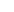with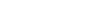which is independent ofand. Defineas,For testing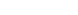against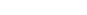consider the test:

Rejectsif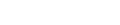.

Find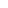such that the test has size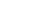.

### Prerequisites

Normal Distribution

Simple Hypothesis Testing

Bernoulli Trials

## Solution :

These problem is simple enough, the only trick is that to observe that the test rule is based on 3 random variables,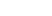andbuton extension is dependent on the the other bernoulli variable.

So, here it is given that we rejectat sizeifsuch that,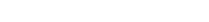So, Using law of Total Probability as,is conditioned on,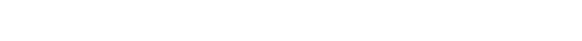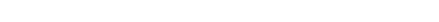[ remember,, andare independent of].

Now, under,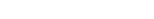and,

So, the rest part is quite obvious and easy to figure it out which I leave it is an exercise itself !!

## Food For Thought

Lets end this discussion with some exponential,

Suppose,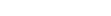are a random sample from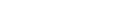and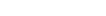is another random sample from the population of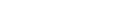. Now you are to test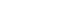against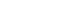.

Can you show that the test can be based on a statistic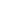such that,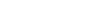.

What distribution you think, T should follow under null hypothesis ? Think it over !!

## Subscribe to Cheenta at Youtube

This is a very simple and beautiful sample problem from ISI MStat PSB 2013 Problem 7. It is mainly based on simple hypothesis testing of normal variables where it is just modified with a bernoulli random variable. Try it!

## Problem- ISI MStat PSB 2013 Problem 7

Supposeandare two independent and identically distributed random variables with. Further consider a Bernoulli random variablewithwhich is independent ofand. Defineas,For testingagainstconsider the test:

Rejectsif.

Findsuch that the test has size.

### Prerequisites

Normal Distribution

Simple Hypothesis Testing

Bernoulli Trials

## Solution :

These problem is simple enough, the only trick is that to observe that the test rule is based on 3 random variables,andbuton extension is dependent on the the other bernoulli variable.

So, here it is given that we rejectat sizeifsuch that,So, Using law of Total Probability as,is conditioned on,[ remember,, andare independent of].

Now, under,and,

So, the rest part is quite obvious and easy to figure it out which I leave it is an exercise itself !!

## Food For Thought

Lets end this discussion with some exponential,

Suppose,are a random sample fromandis another random sample from the population of. Now you are to testagainst.

Can you show that the test can be based on a statisticsuch that,.

What distribution you think, T should follow under null hypothesis ? Think it over !!

## Subscribe to Cheenta at Youtube

This site uses Akismet to reduce spam. Learn how your comment data is processed.

### Knowledge Partner